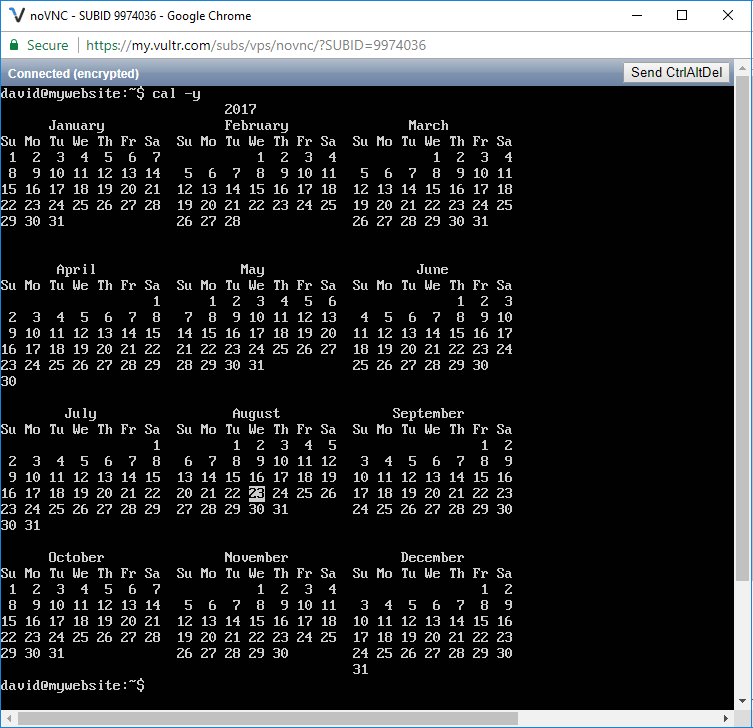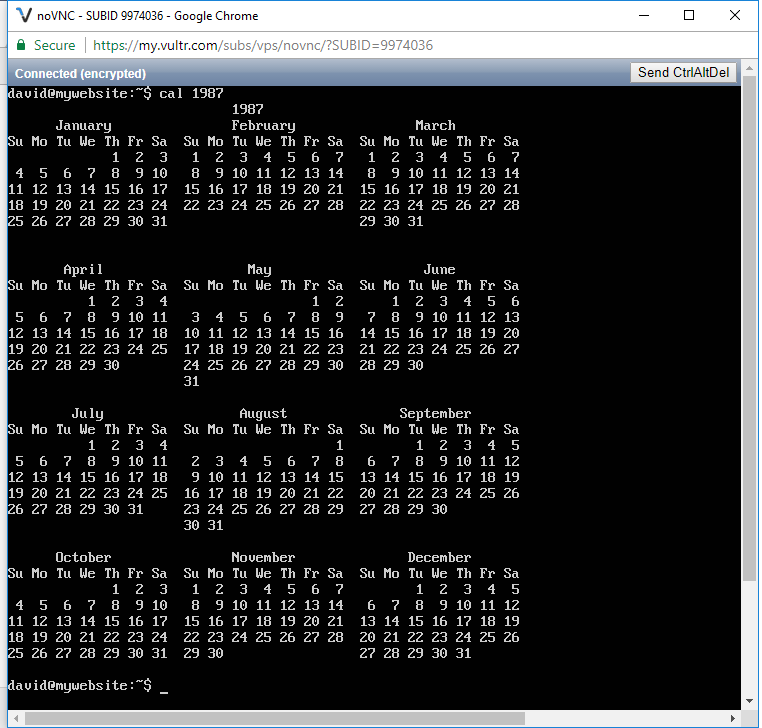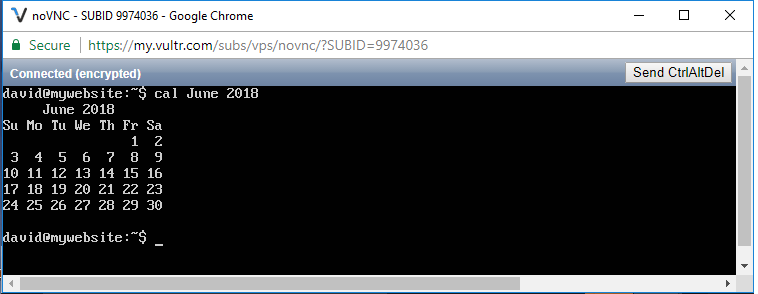﻿ How to Display a Calendar in Linux ﻿# How to Display a Calendar in LinuxIn this article, we show how to display a calendar in linux.

So we can show a calendar in the command line of a linux terminal.

And we show a calendar with the keyword, cal

Now there's a few ways we can display a calendar in linux.

If we simply put the word, cal, without anything else, then we'll get the current month.

So if we just put the following keyword below, we get the calendar for the current month we are in.

Running the keyword above gives us the following output shown below.Next, if we want to show the entire calendar for the current year, we specify the following below.

The following code above will show us the full calendar for the current year.So you see the full year calendar for the current year.

We can show the calendar for any year in linux. We do this by specifying, cal followed by the year.

The following code below shows us the calendar for the year 1987.

Running this code, we get the following output shown below.We can also display just the month for any given month of any year in linux.

We can specify the calendar for whatever month we want to view with the line, cal followed by the month and year of that time.

So with the following code below, we show the calendar for June of 2018.

So with this code, the calendar for June of 2018 will be displayed.

This is shown below.And this is how we can display a calendar in linux.

Related Resources

How to Randomly Select From or Shuffle a List in Python